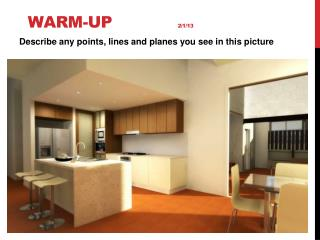DownloadDownload PresentationWarm-Up 2/1/13

# Warm-Up 2/1/13

Télécharger la présentation## Warm-Up 2/1/13

- - - - - - - - - - - - - - - - - - - - - - - - - - - E N D - - - - - - - - - - - - - - - - - - - - - - - - - - -
##### Presentation Transcript

1. Warm-Up 2/1/13 Describe any points, lines and planes you see in this picture

2. Unit 2: Introduction to Geometry • Essential Question: • How do the basic properties of geometry apply to the real world? How do you use deductive reasoning, logic, and mathematical properties to help you draw conclusions? • Bonus Question: • Write real-world examples of conditionals and converses that meet the following criteria, and explain each answer. • A) Write a conditional that is true but whose converse is false. • B) Write a true conditional whose converse is also true.

3. Point Points, Lines, and Planes Definition: A point is an indication of a location. A point has no size. A point is represented by a small dot and is named by a capital letter Example: Name: Point A A

4. Line Definition: A line is a series of points that extends in two opposite directions without end. You can name a line by any two points on the line. Another way to name a line is with a single lowercase letter. Names: **must be only two points**

5. Collinear points Definition: Collinear points are points that lie on the same line. In the picture below, points A and B are collinear but C is not Name the collinear points: B A C

6. Plane Definition: A plane is a flat surface that has no thickness. A plane contains many lines and extends without end in the directions of all its lines. You can name a plane by either a single capital letter or by at least three of its non-collinear points. Vertical • Horizontal Plane Plane A C B P

7. Plane • Names:

8. Coplanar Definition: Points and lines in the same plane are coplanar. M P

9. Naming a Plane

10. Space: Space is the boundless, three-dimensional set of all points

11. Intersection Intersection: the set of points that two figures have in common.

12. Basic Postulates of Geometry (A Postulate is a rule accepted as true without proof) Draw two points and connect the points. What is the geometric figure.

13. Postulate 1-1 • Through any two points there is exactly one line. • Line t is the only line that passes through points A and B. t B A

14. Postulate 1-2 • If two lines intersect, then they intersect in exactly one point • AE and BD intersect at C A B C D E

15. What is the intersection of the front wall and the ceiling?

16. Postulate 1-3 • If two planes intersect, then they intersect in exactly one line. • Plane RST and plane STW intersect in ST R T S W

17. Postulate 1-4 • Through any three noncollinear points there is exactly one plane

18. a) Name the plane on the bottom of the box • b) Shade the plane that contains E, H, and C. • c) Name another point that is in the same plane of point A, B, and C. • d) Name another point that is coplanar with points E, H, and C. • e) Are points A and G collinear?

19. 2. Name the intersection of… A. Planes HGF and GCB GF B. Planes HDC and DAB DC C. Planes EHD and FGC None

20. d. Plane EFB and Point B e. Plane HEB and Point C f. Plane HEF and Point F

21. Line Segment Line Segments, Rays, Parallel Lines and Planes • Definition: A segment is a part of a line consisting of two endpoints and all points between • Names: or

22. Ray • Definition: A ray is the part of a line consisting of one endpoint and all the points of the line on one side of the endpoint.

23. Opposite Rays

24. Name three segments. • Name four different rays. • Name two opposite rays.

25. What are Parallel Lines? Are you sure you have the correct definition? Definition: Parallel Lines are coplanar lines that do not intersect What other type of lines do not intersect????

26. Skew Lines Definition: Skew Lines are noncoplanar lines that do not intersect Are there any in the classroom?

27. Name the segments parallel to: Name a pair of skew lines.

28. Parallel Planes: Parallel Planes: planes that do not intersect. EX. Top ll bottom HGFE ll DCBA

29. Name a pair of parallel planes.

30. Homework • 1-3 Segments, Rays, Parallel Lines and Planes Worksheet • Quiz Wednesday!